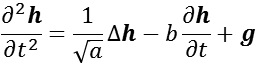Fast and Exact (Poisson) Solvers on Symmetric Geometries

links description executable user interface notes

SGP 2015 Paper
Windows Executables
Source Code
DLLs

CODE DESCRIPTION
This distribution contains the executables for three applications requiring the solution of the Poisson equation on surfaces of revolution:
• Stitching: Given an input image consisting of composited images, and given a mask which assigns the same color ID to pixels coming from the same source image, our code outputs the stitched image whose gradients are a best-fit to the gradients of the composite image, with forced zero gradients across seam boundaries.
• Smoothing/Sharpening/High-Low Compositing: Given input low- and high-frequency images, a gradient modulation term, and a pixel fidelity term, our code outputs the best-fit image whose gradients match the modulated gradients of the high-frequency input image and whose pixel values match the pixel values of the input low-frequency image. Formally, if f1 is the low-frequency image, f2 is the high-frequency image, α is the low-frequency fidelity term, and β is the gradient modulation term, the output image G is the image minimizing the energy:• SoRFlowVisualization: A visualization of incompressible fluid simulation on surfaces of revolution.
The solver follows Stam's stable fluid approach for solving the Navier-Stokes equation:where u is the velocity of the flow, ν is the kinematic viscosity, and g is an external force.
• SoRWaveVisualization: A visualization of wave propagation on surfaces of revolution.
The solver solves performs implicit time-integration to advance the wave given by:where h is the height of the wave, a is the wave speed, b is the damping coefficient, and g is an external force.
All three applications require the FFTW libraries. The two visualization applications require the GLUT libraries.
The source includes both a Visual Studios solution file and a Makefile.
The code also requires installation of the zlib, png, tiff, and jpg libraries to support image I/O. Source code for these is included for compilation using Visual Studios. The Makefile assumes that the header files can be found in /usr/local/include/ and that the library files can be found in /usr/local/lib/.

EXECUTABLE ARGUMENTS
--pixels <input (high-frequency) image>
This string is the the name of the image file containing the (high-frequency) input. Because the FFT is performed along the rows of the image (parallels of the sphere), the computation is faster when this is a power of two. (Currently supported file-types include PNG and JPEG.)
[--lowPixels <input low-frequency image>]
This optional string is the the name of the image file containing the low-frequency input. The resolution of this image must match the resolution of the high-frequency content. If this file is not specified, the argument to --pixels is used for both low- and high-frequency content.
This optional string is the name of the image file serving as the mask for stitching. (Since the values of the mask are used to determine if adjacent pixels in the composite image come from the same source, the mask should not be compressed using lossy compression. Similarly, in representing the composited pixels, be wary of using JPEG compression. Even at 100% quality, it can blur out the seams between images, so that setting the seam-crossing gradient to zero is no longer sufficient.)
This parameter is required when performing stiching, as it lets the system know where to set the seam-crossing gradients to zero.
--out <ouput image>
This string is the name of the image to which the output will be written.
[--iWeight <pixel fidelity term>]
If the system is solving the Poisson equation to perform image smoothing or sharpening, this value specifies the fidelity term α.
If the system is solving the Poisson equation to perform image smoothing or sharpening, this value specifies the gradient modulation β.
This optional integer specifies the number of threads the client should spawn in order to solve its part of the problem.
[--single]
If this optional argument is specified floating point calculations will be performed using single-precision. Otherwise, double-precision floats will be used.
• SoRFlowVisualization and SoRWaveVisualization
--res <azimuthal sampling resolution>
This integer specifies the resolution at which the parallels are to be sampled. Because this is direction along which the FFT is performed, performance is better when this is a power of two.
[--cRes <elevational sampling resolution>]
This optional integer specifies the resolution at which the meridians are to be sampled. If it is not specified, the azimuthal sampling resolution is used.
This optional integer specifies the number of threads the client should spawn in order to solve its part of the problem.
[--single]
If this optional argument floating point calculations will be performed using single-precision. Otherwise, double-precision floats will be used.

USER INTERFACE (SoRFlowVisualization and SoRWaveVisualization)
Both of these executables start off by displaying a curve editing window, allowing the user to specify the generating curve for the surface of revolution. Then, the user switches to the surface viewer and interacts with the simulations.
• Curve Editing Window
Keyboard:
[Esc]: Quit
[Tab]: Switch to simulation window
c: Toggle curve type
a: Specify angle of revolution (in degrees)
i: Save frame buffer to file
h: Toggle help
H: Toggle information
b [SoRWaveVisualzation]: Toggle boundary types
Mouse:
[LEFT]: Drag a control point, insert a new control point along the edge between two existing points, add a new control point.
Note that the interior of the generating curve should not cross the y-axis as this will result in a singularity on the surface.

• Simulation Window
Keyboard: (Note that this is only a subset of the keyboard controls. For a full list, see the help menu on the bottom right.)
[Esc]: Quit
[Tab]: Switch to simulation window
[Space]: Pause simulation
a/z: Rotate around the x-axis
q/w: Rotate around the y-axis
s/x: Rotate around the z-axis
R: Reset simulation
v [SoRFlowVisualization]: Toggle velocity field
r [SoRWaveVisualization]: Toggle rain
Mouse:
[LEFT]+[Ctrl]: Rotate around the x- and y-axes
[RIGHT]+[Ctrl]: Zoom in and out
[LEFT]/[RIGHT]+[Shift] [SoRFlowVisualization]: Inject flow and ink